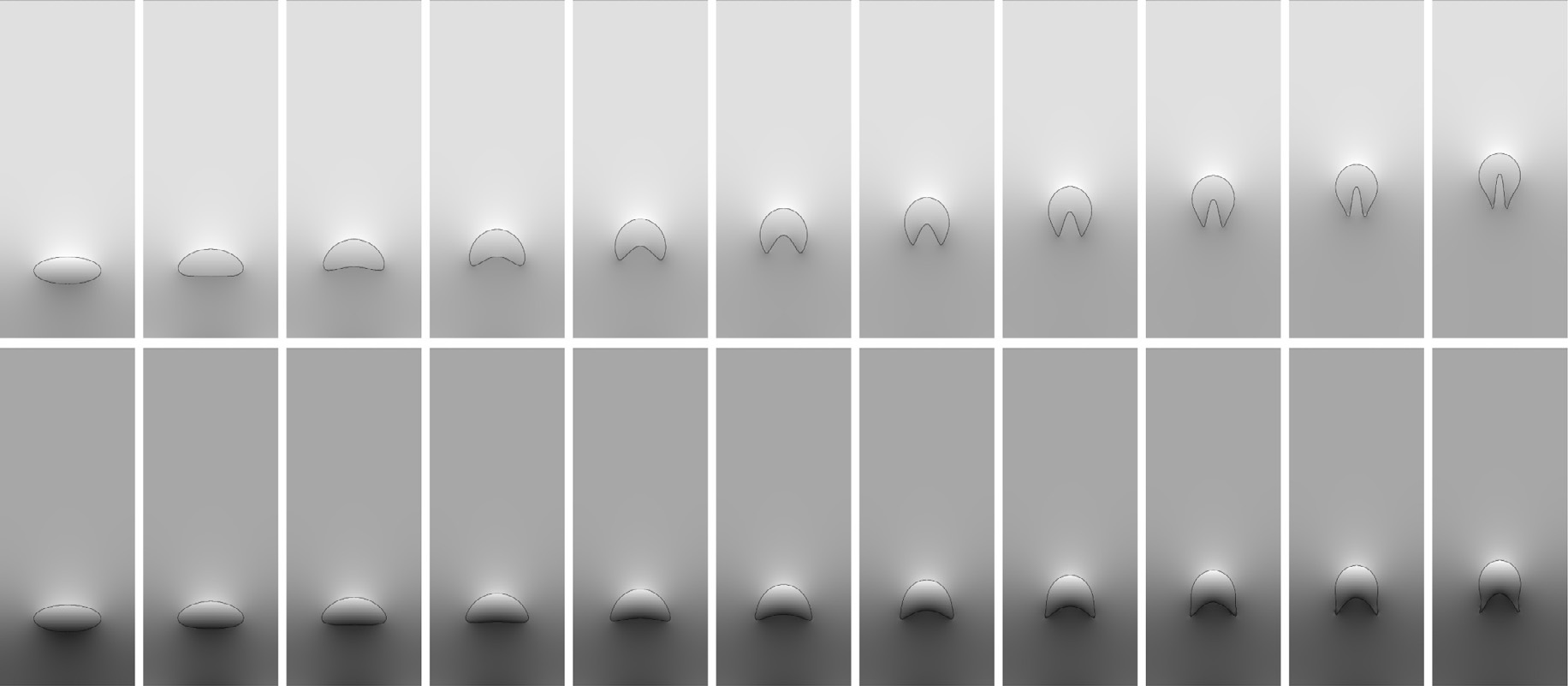A Second Order Virtual Node Algorithm for Navier-Stokes Flow Problems with Interfacial Forces and Discontinuous Material PropertiesC. Schroeder, A. Stomakhin, R. Howes and J. Teran.  A Second Order Virtual Node Algorithm for Navier-Stokes Flow Problems with Interfacial Forces and Discontinuous Material Properties.  Journal of Computational Physics 2014.

We present a numerical method for the solution of the Navier-Stokes equations in three dimensions that handles interfacial discontinuities due to singular forces and discontinuous fluid properties such as viscosity and density. We show that this also allows for the enforcement of normal stress and velocity boundary conditions on irregular domains. The method provides treatment of fluid inertia as well as a new discretization of jump and boundary conditions that accurately resolves null modes in both two and three dimensions. We discretize the equations using an embedded approach on a uniform MAC grid to yield discretely divergence-free velocities that are second order accurate. We maintain our interface using the level set method or, when more appropriate, the particle level set method. We show how to implement Dirichlet (known velocity), Neumann (known normal stress), and slip velocity boundary conditions as special cases of our interface representation. The method leads to a discrete, symmetric KKT system for velocities, pressures, and Lagrange multipliers. We also present a novel simplification to the standard combination of the second order semi-Lagrangian and BDF schemes for discretizing the inertial terms. Numerical results indicate second order spatial accuracy for the velocities (L-inf and L2) and first order for the pressure (in L-inf, second order in L2). Our temporal discretization is also second order accurate.